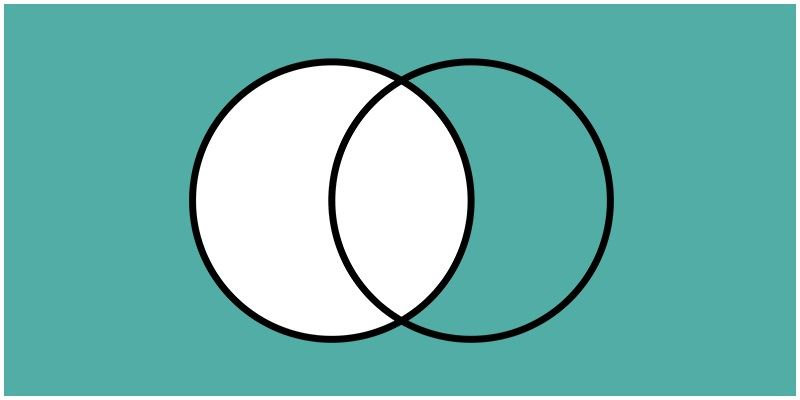# Complement of a Set

Before studying about the Complement of a set, let us understand what are sets?

## Sets Definition

A well-defined collection of objects or elements is known as a set. Any set consisting of all the objects or elements related to a particular context is defined as a universal set. It is represented by U.
For any set A which is a subset of the universal set U, the complement of the set A consists of those elements which are the members or elements of the universal set U but not of the set A. The complement of any set A is denoted by A’.

## Complement of a Set Definition

If U is a universal set and A be any subset of U then the complement of A is the set of all members of the universal set U which are not the elements of A.

$$\begin{array}{l}A’ = {x ~:~ x~ ∈ ~U ~and ~x ~∉ ~A}\end{array}$$

Alternatively it can be said that the difference of the universal set U and the subset A gives us the complement of set A.

## Venn Diagram for the Complement of a set

The Venn diagram to represent the complement of a set A is given by:### Complement of a Set Examples

To make it more clear consider a universal set U of all natural numbers less than or equal to 20.

Let the set A which is a subset of U be defined as the set which consists of all the prime numbers.

Thus we can see that A = {{2, 3, 5, 7, 11, 13, 17, 19}}

Now the complement of this set A consists of all those elements which is present in the universal set but not in A. Therefore, A’ is given by:

A’={{1,4,6,8,9,10,12,14,15,16,18,20}}

Example: Let U be the universal set which consists of all the integers greater than 5 but less than or equal to 25. Let A and B be the subsets of U defined as:

$$\begin{array}{l}A= {{x~:x~ ∈~U ~and~ x~ is~ a~ perfect~ square}}\end{array}$$

B = {7, 9, 16, 18, 24}

Find the complement of sets A and B and the intersection of both the complemented sets.

Solution: The universal set is defined as:

U = {{6,7,8,9,10,11,12,13,14,15,16,17,18,19,20,21,22,23,24,25}}

Also, A = {9,16,25} and

B = {7,9,16,18,24}

The complement of set A is defined as:

$$\begin{array}{l}A’ = {x~:~x~∈~U~ and ~x~∉~A}\end{array}$$

Therefore,

A’ = {6,7,8,10,11,12,13,14,15,17,18,19,20,21,22,23,24}

Similarly the complement of set B can be given by:

B’ = {6,8,10,11,12,13,14,15,17,19,20,21,22,23,25}

The intersection of both the complemented sets is given by A’∩ B’.

Rightarrow A’∩ B’= {6, 8, 10, 11, 12, 13, 14, 15, 17, 19, 20, 21, 22, 23}

We can see from the above discussions that if a set A is a subset of the universal set U then the complement of set A, i.e. A’ is also a subset of U.

## Video Lesson on What are SetsNow we are clear on the concept of the complement of sets. There is a lot more to learn. Enrich your knowledge and reach new horizons of success by downloading BYJU’S – The Learning App to know more or visit our website.

Test your knowledge on Complement Of Set